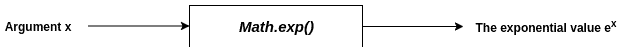# JavaScript Exponential: What is Math.exp() Function

JavaScript Math.exp() is a built-in method that returns the value of ex, where e is Euler’s number (approximately 2.7183), and x is the number passed to it. It is a static method of Math, so you always use it as Math.exp(), not as a method of a Math object.

## JavaScript exponential

To find an exponential value in JavaScript, you can use the Math.exp() method. The Math.exp() function returns ex, where x is an argument, and e is the Euler’s number, a base of the natural logarithms.

### Syntax

``Math.exp(x)``

### Parameter(s)

The variable x, whose exponential value is ex, must be determined.

### Return Value

The exponential value ex.

### Visual representation#### Note

1. For a positive argument x, Math.log(Math.exp(x)) gives the value x.
2. If the argument is negative infinity, then this method returns 0.
3. If the argument is positive infinity, then this method returns positive infinity.
4. If an argument is positive zero or negative zero, then this method returns 1.
5. If the passed value is not a valid number, the method returns NaN.
6. If the passed value is null, the method returns 1.

### Example 1

``````var a = 1;
var b = -1;
var c = 2;
var d = 3;

console.log(Math.exp(a));
console.log(Math.exp(b));
console.log(Math.exp(c));
console.log(Math.exp(d));``````

Output

``````2.718281828459045
0.36787944117144233
7.38905609893065
20.085536923187668``````

### Example 2

``````var a = "JavaScript";
var b;

console.log(Math.exp(a));
console.log(Math.exp(b));``````

Output

``````NaN
NaN``````

### Example 3

JavaScript exp() method cannot be used with complex arguments, as only integer arguments are accepted.

``console.log(Math.exp(2+i));``

#### Output

``ReferenceError: i is not defined``

### Example 4

The following example demonstrates that for a positive x: ln(ex) = x.

``````var x = 10;

var a = Math.exp(x);

console.log(Math.log(a));``````

Output

``10``

### Example 5

The following example demonstrates the case of the parameter as infinity(positive/negative) and zero(positive/negative).

``````var a = Number.POSITIVE_INFINITY;
var b = Number.NEGATIVE_INFINITY;

var c = 0;
var d = -0;

console.log(Math.exp(a));
console.log(Math.exp(b));
console.log(Math.exp(c));
console.log(Math.exp(d));``````

Output

``````Infinity
0
1
1
``````

### Example 6

The following example demonstrates that if null is passed as an ent, this method returns 1.

``````var a = null;
var b = "";
var c = [];

console.log(Math.exp(a));
console.log(Math.exp(b));
console.log(Math.exp(c));``````

Output

``````1
1
1``````

### Example 7

The following example demonstrates the application of this method in a simple programming context.

Given any x, compute the value of the infinite series:

1 + x/1! + x2/2! + x3/3! + x4/4! + …

[Now, it would be tough to devise a program to solve this. But since

1 + x/1! + x2/2! + x3/3! + x4/4! + …  = ex,

It can be done within a few lines of code].

``````var x;

const rl = r.createInterface({
input: process.stdin,
output: process.stdout
});

const prompt1 = () => {
return new Promise((resolve, reject) => {
resolve();
});
});
};

const main = async () => {
await prompt1();
rl.close();
console.log();
console.log('The value of the series 1 + x/1! + (x^2)/2! + (x^3)/3! + ... :');
console.log(Math.exp(x));
}
main();``````

Output

``````Test Case 1:
x: 1

The value of the series 1 + x/1! + (x^2)/2! + (x^3)/3! + ... :
2.718281828459045

Test Case 2:
x: 2

The value of the series 1 + x/1! + (x^2)/2! + (x^3)/3! + ... :
7.38905609893065``````

That’s it.

### Compatibility(Version and above)

2. Internet Explorer v3
3. Firefox v1
4. Edge v12
5. Opera
6. Safari v1
7. Android webview v1
8. Chrome for Android v18
9. Firefox for Android v4
10. Opera for Android
11. Safari on iOS v1
12. Samsung Internet v1.0
13. Node.js

JavaScript version: ECMAScript 1

That’s it.

This site uses Akismet to reduce spam. Learn how your comment data is processed.